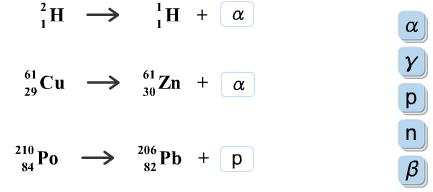# Problem: Complete these nuclear reactions.

###### FREE Expert Solution

Recall that in a nuclear reaction, the number of protons and neutrons is affected and the identity of the element changes

The different types of radioactive decay are:

• Alpha decay: forms an alpha particle (42α, atomic mass = 4, atomic number = 2)

• Beta decay: forms a beta particle (0–1β, atomic mass = 0, atomic number = –1). The beta particle appears in the product side.

• Gamma emission: forms a gamma particle (00γ, atomic mass = 0, atomic number = 0)

• Positron emission: forms a positron particle (01e, atomic mass = 0, atomic number = 1)

• Electron capture: the initial nuclide captures an electron (0–1e, atomic mass = 0, atomic number = –1). The electron appears in the reactant side.
• Neutron10n, atomic mass = 1, atomic number = 0

The nuclear equation must be balanced with the same total atomic mass and atomic number on both sides.###### Problem Details

Complete these nuclear reactions.